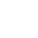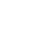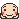LV. 50
GP 8k1 記得這編不到三個月前的文嗎?^^^^^^^^^^^^^^^^^^^^^^^^^^^^^^^^^^^^^^^^^^^

https://forum.gamer.com.tw/C.php?bsn=60037&snA=72931&tnum=10

2 為什麼超能力者這麼白痴?為本編護航
^^^^^^^^^^^^^^^^^^^^^^^^^^^^^^^^^

3 為什麼熱愛這部作品?
^^^^^^^^^^^^^^^^^^^^^^^^^

4 上次的討論串中沒有人說過的最新發展和細節
^^^^^^^^^^^^^^^^^^^^^^^^^^^^^^^^^^^^^^^^^^^^^

5 看到這里的感想和評價
^^^^^^^^^^^^^^^^^^^^^^

face基於日前微軟官方表示 Internet Explorer 不再支援新的網路標準，可能無法使用新的應用程式來呈現網站內容，在瀏覽器支援度及網站安全性的雙重考量下，為了讓巴友們有更好的使用體驗，巴哈姆特即將於 2019年9月2日 停止支援 Internet Explorer 瀏覽器的頁面呈現和功能。